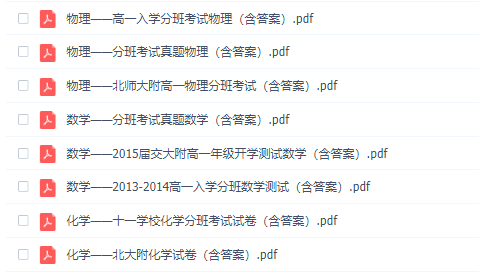# 新高一分班考试数学试卷原题与解析，快来看看

2020-08-02 19:59:37 　来源：网络整理点击领取>>>2016-2020北京各高中开学高一分班考试试卷&各学科思维导图&期中试卷及解析

新高一分班考试数学试卷原题与解析，快来看看！看看往年的高一分班考试卷吧，然后做好复习，迎接考试，加油~~下面小编就给大家带来新高一分班考试数学试卷原题与解析，快来看看，希望对大家有所帮助哦！

1 . 适用条件

[直线过焦点]，必有ecosA=(x-1)/(x+1)，其中A为直线与焦点所在轴夹角，是锐角。x为分离比，必须大于1。

注：上述公式适合一切圆锥曲线。如果焦点内分(指的是焦点在所截线段上)，用该公式;如果外分(焦点在所截线段延长线上)，右边为(x+1)/(x-1)，其他不变。

2 . 函数的周期性问题(记忆三个)

(1)若f(x)=-f(x+k)，则T=2k;

(2)若f(x)=m/(x+k)(m不为0)，则T=2k;

(3)若f(x)=f(x+k)+f(x-k)，则T=6k。

注意点：a.周期函数，周期必无限b.周期函数未必存在最小周期，如：常数函数。c.周期函数加周期函数未必是周期函数，如：y=sinxy=sin派x相加不是周期函数。

3 . 关于对称问题(无数人搞不懂的问题)总结如下

(1)若在R上(下同)满足：f(a+x)=f(b-x)恒成立，对称轴为x=(a+b)/2

(2)函数y=f(a+x)与y=f(b-x)的图像关于x=(b-a)/2对称;

(3)若f(a+x)+f(a-x)=2b，则f(x)图像关于(a，b)中心对称

4 . 函数奇偶性

(1)对于属于R上的奇函数有f(0)=0;

(2)对于含参函数，奇函数没有偶次方项，偶函数没有奇次方项

(3)奇偶性作用不大，一般用于选择填空

5 . 数列爆强定律

(1)等差数列中：S奇=na中，例如S13=13a7(13和7为下角标);

(2)等差数列中：S(n)、S(2n)-S(n)、S(3n)-S(2n)成等差

(3)等比数列中，上述2中各项在公比不为负一时成等比，在q=-1时，未必成立

(4)等比数列爆强公式：S(n+m)=S(m)+q²mS(n)可以迅速求q点击领取：《2016-2020北京各高中开学高一分班考试试卷&各学科思维导图&期中试卷及解析》

部分资料截图如下：QQ扫一扫您将获得群内福利家长互动海量资料同步免费视频课程了解最新信息及时解读资讯你可能感兴趣的文章立即咨询1对1在线课程*学而思爱智康会在24小时内与您取得电话联系大家都在看热门课程介绍限时免费领取学习相关

• 全国课程在线咨询
• 咨询热线：4000-121-121
• 扫描注册有礼

• 让学习更有效